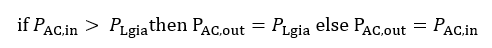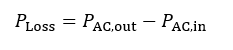# AC Subsystem

Once the output (voltage, current, and power) of a DC array has been computed, the further downstream losses, including inverter, transformer, auxiliary, and other losses, are modeled.

A basic outline of the AC subsystem losses appears below:

1. Find the maximum power point of the DC array, and the corresponding voltage and current.

2. Compute the energy lost due to Joule heating in the DC wiring connecting the modules to the inverter. This is a function of the array’s MPP operating current.

3. Compute the energy lost in the inverter due to off-MPP tracking and inverter efficiency.

4. Compute the AC-side model energy loss due to system degradation (modeled at the output of the inverter)

5. Compute the energy consumed by the PCS auxiliary loads (lighting, DAS), and the PCS or inverter cooling

6. Compute the energy lost in the MV transformer.

7. Compute the losses in the aggregate AC cabling between the MV Transformer and the input to the HV transformer.

8. Compute the energy lost in the HV transformer.

9. Compute the energy lost in the transmission line to the point of interconnection.

10. Compute the energy lost due to Array output control to avoid exceeding the plant output limit.

# Elevation and Temperature Derate

Inverters are typically rated in units of kVA at a nominal ambient temperature (typically 50 °C) and at sea level. Maximum inverter kVA capacity can vary with ambient temperature and elevation. It increases at lower temperatures and elevations, and decreas at higher temperatures and elevations. This algorithm calculates the actual maximum AC operating kVA given the ambient temperature, and elevation. An example of the elevation and temperature derate curves is shown in Figure 40.## Inputs## Outputs## Algorithm

1.) Find the inverter apparent power, for the given ambient temperature Ta and site elevation h, by linearly interpolating the tabular inverter derate breakpoints along the ambient temperature. There is only interpolation along the ambient temperature axis, not along the elevation.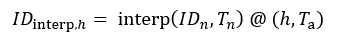For Example: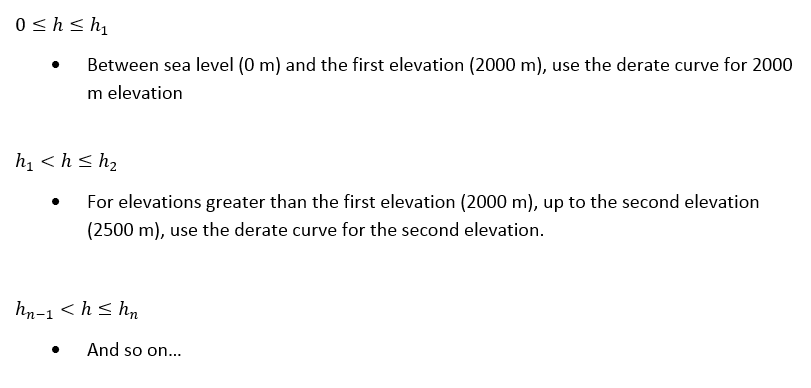2.) Determine if the set point or calculated apparent power governs maximum real power in the inverter operation. This is the lesser of the desired setpoint Pset or the maximum available temperature adjusted apparent power the inverter can produce.

Switch on Inverter Model selection

Case: Temperature Derate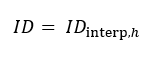Case: None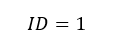Find the inverter clipping limit: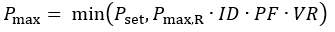# Array Bias (MPP & off-MPP operation)

This function finds the array’s operating point at the array’s nominal (maximum power point) voltage and current bias conditions. Should the MPP fall outside these ranges, the following off-MPP tracking behavior is invoked in an attempt to maintain inverter operation. The inverter operation is divided into 12 zones in the voltage-power space, as show in Figure 41.

The concept of “zone transition” is an artifact of the simulation model, and does not necessarily reflect the transitions of the inverter’s software finite state machine in response to array stimulus. PlantPredict calculates the array bias (voltage & current) at maximum power to determine the “initial zone”. If it happens to be in Zone 6, the MPPT operating window, no further action is taken, and the array bias is set for the current simulation time step.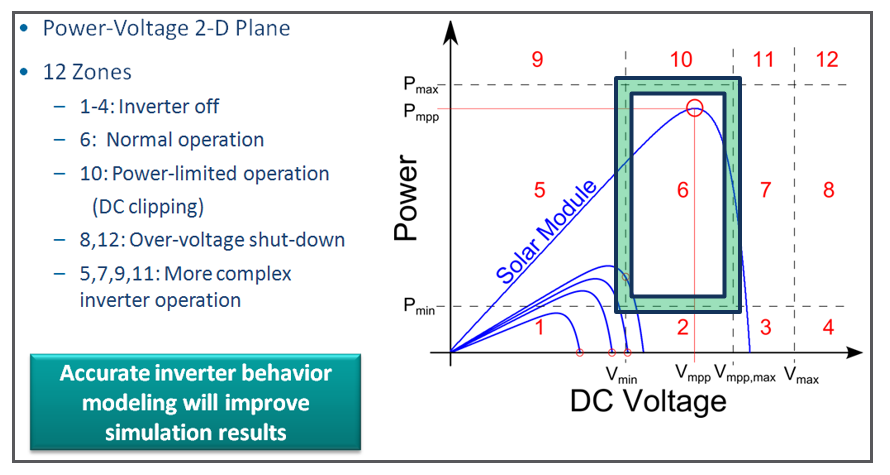### Figure 41. Inverter Operating Window in the Power-Voltage Space, showing 12 Zones of Operation; the Y-axis is AC power

If the array maximum power point falls outside of Zone 6, then PlantPredict seeks to raise or lower the voltage (operate off-MPPT) in order to reach one of the zone boundaries on the green rectangle, specifically the intersections of 5 & 6, 10 & 6, 7 & 6. If the PV curve does not intersect the rectangle, then no power is generated: the inverter is effectively shut off.

## Inputs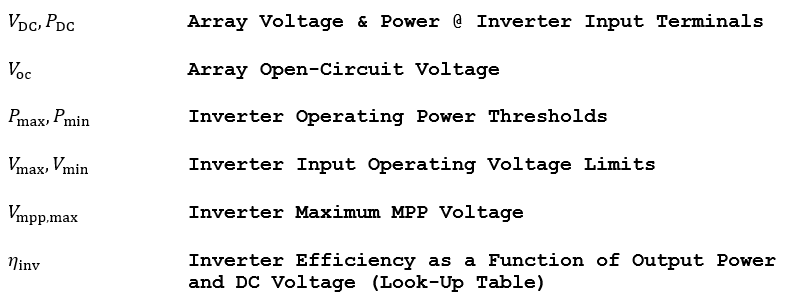## Outputs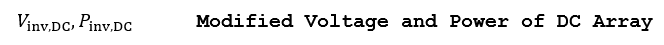## Algorithm

Assume that the inverter is presented with a DC Field biased at VDC to generate DC power, PDC. Verify if the power point falls in one of the following “zones”, and if necessary, modify the array bias, and recheck to see if the power point has changed “zones”. When instructed to check for a zone transition, the following steps should be executed again, with the output set and the procedure exited only if the current “zone” is the same as the “zone” found in the previous iteration.

Zones 1-3: Under-power operation, Pinv,DC < Pmin :

The inverter output is set to zero power. The inverter is shut-off because not enough power can be extracted from the DC Field to operate the inverter’s power electronics. The array remains biased at open-circuit: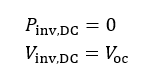Zone 4: Under-power operation & over-voltage operation, Pinv,DC < Pmin & Vinv,DCVmax :

The inverter output is set to zero power. The inverter is shut-off because not enough power can be extracted from the array to operate the inverter’s power electronics. The DC Field is disconnected: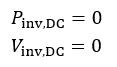Zone 5: Under-voltage operation, Vinv,DCVmaxv :

The inverter has trouble operating because it may not be able to maintain required output voltage due to low input voltage. It will attempt to decrease the current and raise the voltage to reach Vmin; however, if this is not possible, it will set the output power to zero: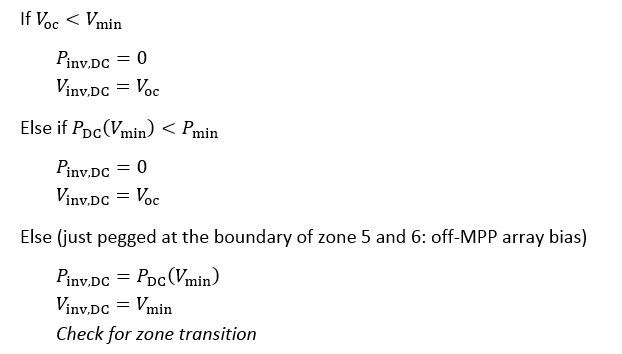Zone 8: Severe over-voltage operation, Vinv,DC > Vmax :

The inverter shuts down and disconnects the DC Field due to over-voltage: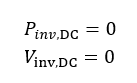Zone 7: Marginal over-voltage operation, Vinv,DC > Vmpp,max :

Increase the current such that voltage drops to Vmpp,max. The operating point slides up the knee of the I(V) curve. Find the new power at Vmpp,max (off-MPP array bias):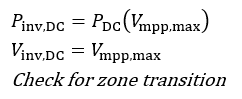Zone 10: Over-power operation Pinv,DC > ηinvPmax :

This is the classic “inverter clipping” case. Raise the inverter voltage in order to reduce the current, such that Pinv,DC = Iinv X Vinv = Pmax. The operating point slides down the knee of the I(V) curve (off-MPP bias):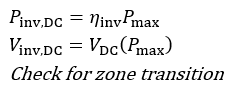Zones 11,12: Over-power operation, Pinv,DC > ηinvPmax & Vinv,DC > Vmpp,max :

This is an extreme over-power and over voltage case. The inverter disconnects the DC Field.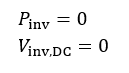Zone 6: Normal operation (fall through case where the maximum power point of the I(V) curve resides in the normal operating window):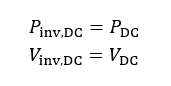# Inverter Efficiency

Compute the inverter output power by applying the inverter efficiency function. The efficiency table lists the inverter’s efficiency as a function of the input power, and at many DC input voltages, as shown in Figure 42.### Figure 42: Example Inverter Efficiency Curves Defined at Low, Medium, and High Voltages

Compute power at the output of the inverter by interpolating the efficiency curve, first by power, then by voltage. An interpolation technique which eliminates any overshoots and ripples, such as a cubic Hermite interpolation, is best, although a linear interpolation will suffice as a first approximation. A linear interpolation (with extrapolation for voltages less than the minimum or greater than the maximum) should be used for the voltages.

PlantPredict currently uses a trilinear interpolation across power and voltage and can support an arbitrary number of efficiency curves, expressed as percentage of AC power at a given input voltage. There is no physical limit on the number of efficiency points per voltage, although typically 3 are provided: one at minimum MPPT voltage, one at nominal voltage, and one at maximum MPPT voltage; furthermore any arbitrary “smoothness” can be achieved by interpolating the CEC, inverter datasheet or factory-witness test efficiency curves using a pre-processed non-linear interpolation.

## Inputs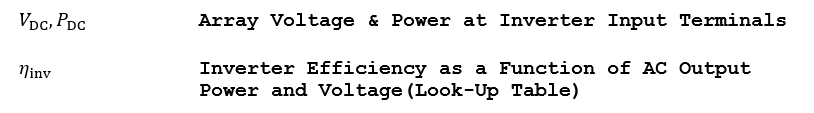## Outputs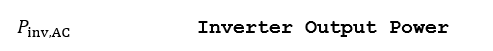## Algorithm

1.) Compute the inverter output power by linearly interpolating the efficiency curve at the DC input power.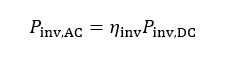# Array and AUX Losses

Compute the losses incurred at the power conversion station. These losses are comprised of parasitic loads for inverter cooling, tracker actuator, and auxiliary loads such as the data acquisition system, lighting, etc.

## Inputs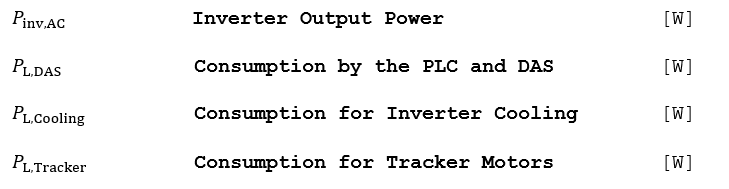## Outputs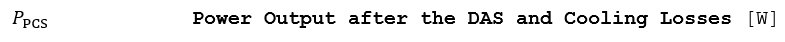## Algorithm

Note the following preconditions before performing the calculations:

a.) The tracker actuator loss PL,Tracker is only applied during daylight hours at Arrays which host one or more tracker DC Fields.

b.) The inverter cooling load loss PL,Cooling is only applied during daylight hours when the inverter is operating.

c.) The auxiliary loss PL,DAS is present for all hours of the day.

1.) Find the power at the input terminal of the Array transformer.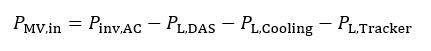2.) Find the power dissipated in the Array transformer.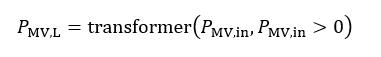3.) Find the power output by the power conversion station.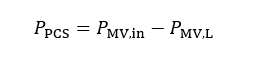# Transformer

Compute the transfer function of the transformer. This function is parameterized to work with any transformer.

## Outputs## Algorithm## Reference

McCann, Lawrence , ABB Electrical Transmission and Distribution Reference, P.101

# AC Collection System

Compute the transfer function of the AC collection system. This loss is a constant proportional loss applied equally at the output of each Array.

## Inputs## Outputs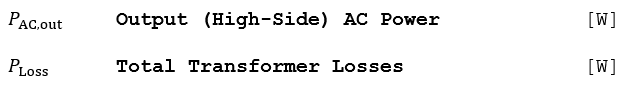## Algorithm

1.) Include flat-rate AC collection loss, only if the power flow is positive.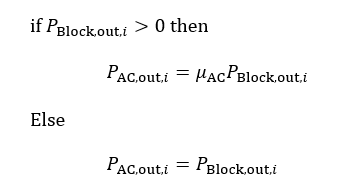2.) Account for the energy dissipated in the lines.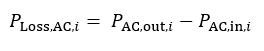# System Transmission Line

This function computes the resistive-loss of a 3-phase transmission line, and does not include the reactive components. This analysis is valid for lines up to 30 miles in length or all line voltages less than 40 kV.

## Inputs## Outputs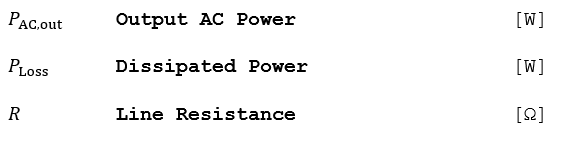## Algorithm

1.) If the input power to the transmission line is negative, set it to zero. This avoids erroneous computations at night.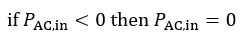2.) Find the total resistance of the line in SI units.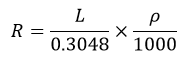3.) Find the phase current.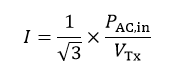4.) Find the power loss in the transmission line.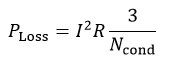5.) Find the power output. If the result is negative, power is being sourced from the grid to energize the transformers (i.e. night-time operation).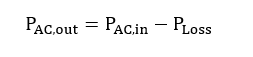Default resistance (“Resistivity”) values are populated in the PlantPredict UI based on the total system AC size, the line voltage and a Phelps-Dodge conductor sizing table.

1.) A required current rating for the line is estimated:2.) For current ratings between 145 Amps and 1200 Amps, the resistance is estimated based on a polynomial fit to the referenced Phelps-Dodge conductor sizing table.3.) Since the polynomial fit was limited to current rating values between 145 Amps and 1200 Amps, current ratings less than 145 Amps are fixed at 0.477 Ohms per 1000 feet and those greater than 1200 Amps are fixed at 0.0165 Ohms per 1000 feet.Individual data points and linear fit to Phelps-Dodge Resistance values as a function of Current Rating.

## Reference

McCann, Lawrence, ABB Electrical Transmission and Distribution Reference, Chapter 9

Phelps-Dodge, Aluminum Conductors, Aluminum-Clad Steel Reinforced. www.pdic.com. PDIC01048. 4/29/05.

# Availability Loss

This function computes the energy lost due to plant downtime by applying the following equation at each time step to the power at the transformer and transmission line output.

## Outputs## Algorithm# Plant Output Control

Compute the transfer function of the plant control loop. This is a very simplified representation of how the output control prevents the plant from delivering more power to the grid than allowed by the interconnection agreement. This routine simply functions as a ceiling on the output.

## Inputs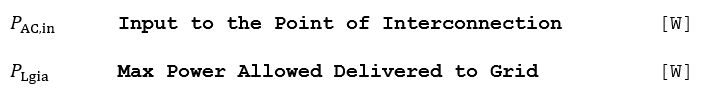## Outputs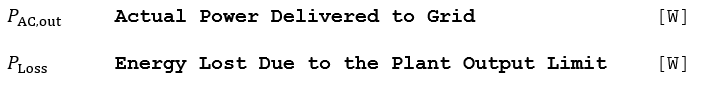## Algorithm

1.) Find the power lost due to AC clipping.